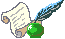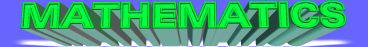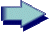MathsScienceRevision GuideLinksArticles

At secondary level mathematics is now split into six main areas of study:

1. Number

2. Algebra

3. Ratio, Proportion & Rates of Change

4. Geometry & Measure

5. Probability

6. Statistics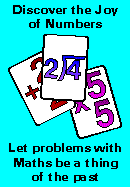Calculators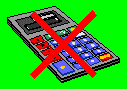With today's Key Stage Three tests and GCSE exams it is very important in being able to carry out basic calculations without the use of a calculator. This requires that you are able to carry out arithmetic using both fractions and decimals.
Using & Applying Mathematics

Although from September 2015 this is not classified as one of the six domains, it is still forms a very important part of mathematics. I will still be showing my students where appropriate the practicle application of the maths being covered. There is no longer a coursework component at G.C.S.E level.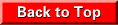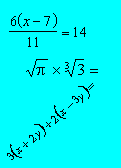Number & Algebra

These two areas of mathematics were formally separate specifications on the curriculum, they are now treated as one specification.

Number at secondary level concerns the ability to carry out calculations using fractions, decimals & percentages.

There are different kinds of number, including fractions, decimals, and whole numbers known as integers. For more details please follow the link shown below.

Number

Algebra is essential to the study of mathematics at all levels beyond primary education, being mainly concerned with solving and forming equations using various rules.

A copy covering the basic rules required for G.C.S.E mathematics can be viewed from the following link.

Rules of Algebra

Ratio, Proportion & Rates of Change

Ratio is concerned with dividing two or more sets of numbers into portions.

Rates of change involves velocity and acceleration, which now include the constant acceleration equation from physics.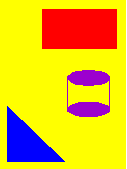Geometry & Measures

Geometry & Measures was formally called Shape, Space & Measures. It is the study of lines and the angular relationship between them, 2D & 3D shapes and their properties. Area, Volume, Similarity and graphs are also included in this specification.

Today there are strong links between geometry and algebra. It is very important that you can rearrange and solve a given formula.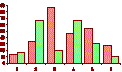Probability & Statistics

Probability & Statistics was formally called Handling. Included in statistics is the representation of data using pie charts, bar charts, histograms and frequency graphs. Also included are the averages the mode, mean and median and their calculation from given data. Probability is concerned with the chance of an event occurring or not occurring, it now includes set theory and the use of Venn diagrams.